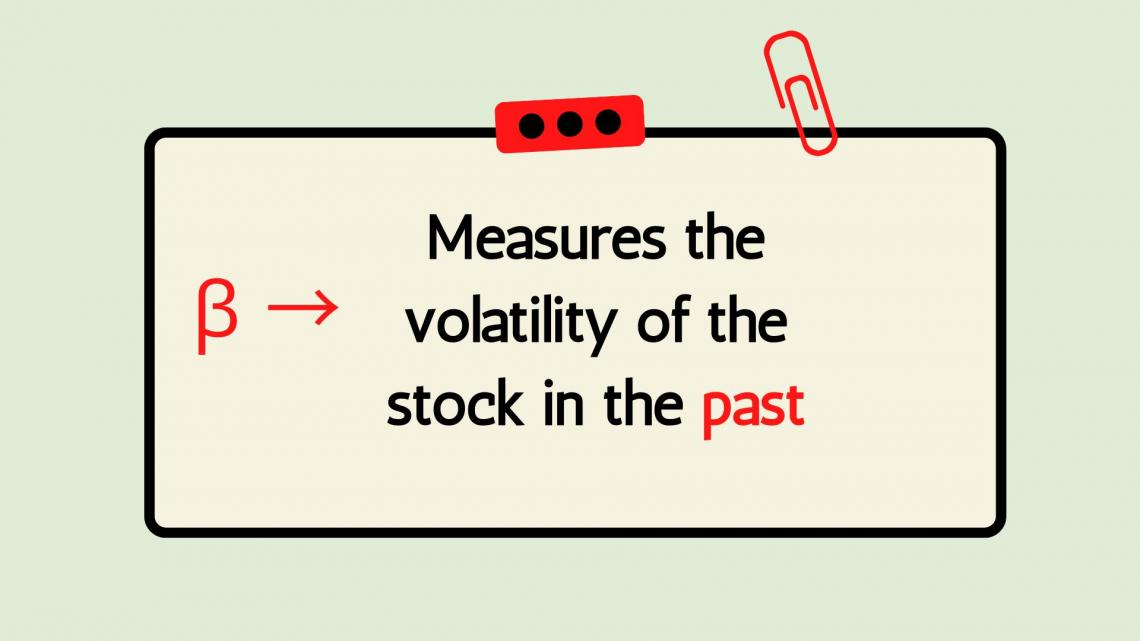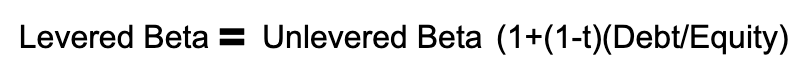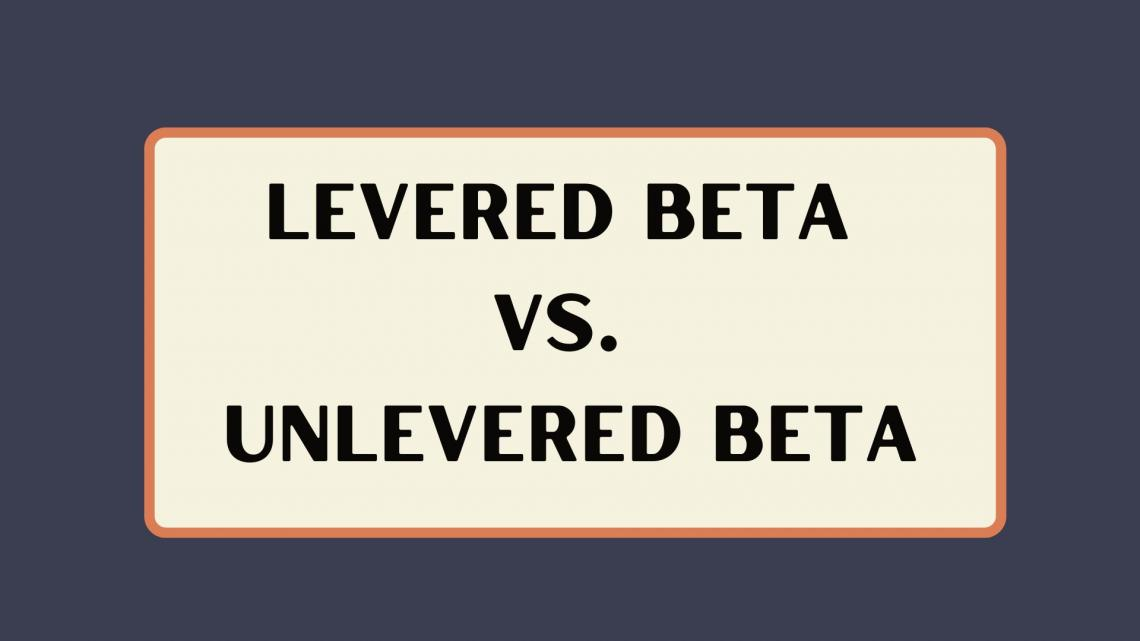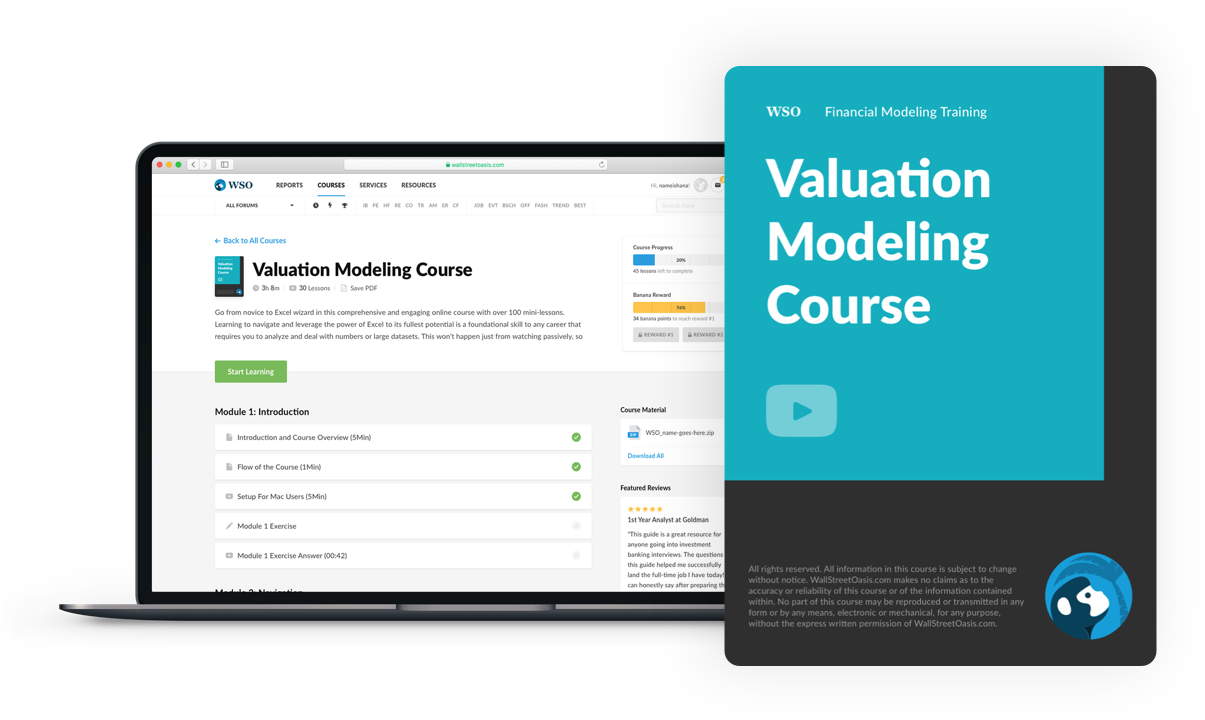# Levered Beta

It is a measure of the risk of a company's stock that is entirely due to macroeconomic changes rather company's decisions

Levered beta measures the risk of a company's capital structure. It is used to compare a company's risk against the market.It is used to understand the systematic risk of a stock. This includes risks that are difficult to predict and adjust for, such as war, natural disasters, and political events.

The equity beta accounts for the entire capital structure of the company. It weighs the risk of the debt-to-equity ratio of a company. Using it can determine the risk of a stock or the risk of your entire portfolio.

Investors use this beta to measure the risk of a company's stock relative to the entire market and/or comparable companies

A beta of 1 means the stock has the same risk as the total market. While a measure above 1 means the stock is riskier than the total market. So, a measure below 1 shows the stock has less risk than the entire market.

## Understanding Beta

Here is how to understand stock betas:

• A beta of 1.0 means the stock moves equally with the market
• A beta of 2.0 means the stock moves twice as much as the market
• A beta of 0.0 means the stock moves do not correlate with the market
• A beta of -1.0 means the stock moves precisely opposite the marketA beta of one means a company has an equal risk to the total market. While a value above one signals the company carries more risk. Oppositely, a value below one signals the company carries less risk.

Investors will use equity beta to compare the risks concerning the total market. But also will compare the beta to competitors in their market.

For example, a company with a beta of 1.2 is considered riskier than the market. But it can be considered a positive sign if it is comparatively lower than other companies.For example, Delta Air Lines has a beta of 1.22. This is not a great mark and says Delta Air Lines is more volatile than the market.

But, some of Delta's largest competitors, JetBlue Airways and United Airlines, both have a beta of 1.39. This makes Delta a much more attractive investment once it is compared to competitors.

Although uncommon, a company can have a negative beta. This means the stock moves opposite to the market. Gold stocks are some of the only securities with negative beta. This includes Barrick Gold ($GOLD), one of the largest gold mining companies. The largest limitation of equity beta is it only relies on past returns. This means only past market performance is included in the beta. As a result, it is impossible to forecast future impacts on returns. Additionally, when making long-term investment decisions, using beta is less useful. Over time the beta could change. This would alter your original initial investment thesis## Formula The formula is :The components of the formula are: • Unlevered Beta(Asset Beta): A company's beta without including debt. Measures the volatility of returns against the market. Only accounts for company assets and the risk associated with them. • t: The tax rate for the company. The tax rate varies based on location, income, and political climate. • Debt: Money borrowed that the company must repay. Companies use debt to make purchases they would be unable to make, given their current financial situation. Debt primarily comes from loans and bonds issued by a company. • Equity: Amount of money that would be returned to shareholders if all assets were liquidated after debts were paid off. Simply, it is left over after subtracting total assets by total liabilities. Equity is also referred to as shareholder's equity. Levered Beta vs Unlevered Beta: The key difference between levered and unlevered beta is the inclusion of debt. The latter only accounts for equity in the capital structure, while the first accounts for debt and equity in the capital structure.Investors commonly use asset beta when deciding to invest in company stock. They use it because it's better to compare companies' risks on an even playing field. Removing the debt allows investors to look only at the company's equity risk. ## Example of Levered Beta Using the following information, calculate the levered beta for Company X: Unlevered Beta: 1.1 Tax Rate: 22% Debt to Equity: .2 To start, subtract the tax rate of 22% by 1. This gives you .78. Then multiply .78 by the debt to equity ratio of .2. This is .156. Then add 1 to get 1.156. Finally, multiply 1.156 by the unlevered beta of 1.1. This gives you 1.2716, which is the levered beta.Extended Example: Using the following information, calculate the levered beta for Company X: Unlevered Beta: .85 Income Before Taxes:$1,000,000

Taxes: $250,000 Debt:$150,000

Equity: \$750,000

Before using the formula, determine the tax rate and debt-to-equity ratio. To find the tax rate divide the taxes by income before taxes. It would be 250,000/1,000,000 which is .25 or 25%. To find the debt to equity ratio, divide debt by equity. It would be 150,000/750,000 or .2.

Now that we have all inputs for the formula, we can use it to find the equity beta.

To start, subtract the tax rate of 25% by 1. This gives you .75. Then multiply .75 by the debt to equity ratio of .2. This is .15. Then add 1 to get 1.15. Finally, multiply 1.15 by the unlevered beta of .85. This gives you .9775, which is the levered beta.

Levered Beta Calculator: A beta calculator can be helpful when attempting to find this measure. Calculator Academy has a free-to-use online levered beta calculator. Use the calculator and plug in asset beta, tax rate, debt, and equity values. Below is what the calculator looks like:## Debt to Equity Ratio

The debt to equity ratio is total liabilities divided by total shareholders' equity. It compares the amount of debt a company has with the total amount of equity. Understanding the debt-to-equity ratios allows you to value the leverage of a company.A "good" debt-to-equity ratio varies in business industry and size. But typically, a ratio below one is considered to be strong. While a ratio above 2 is very risky. It signals the company has a high level of debt. Investing in a company with lots of debt increases the risk associated with the company.

The debt-to-equity ratio is included in the equity beta formula. The higher the debt-to-equity ratio, the higher the leverage beta.

This highlights the point that additional debt will increase the risk of the stock. In addition, debt to equity ratio impacts equity beta as a higher debt-to-equity ratio will make it increase.### Everything You Need To Master Valuation Modeling

To Help You Thrive in the Most Prestigious Jobs on Wall Street.

Reviewed and Edited by Abhijeet Avhale | LinkedIn